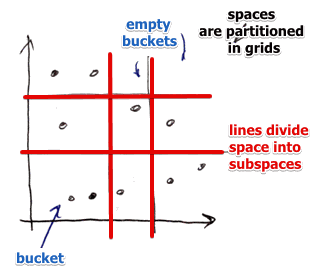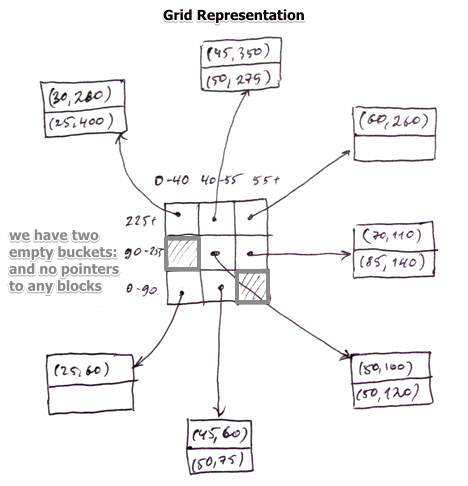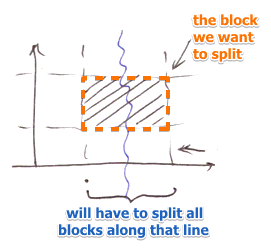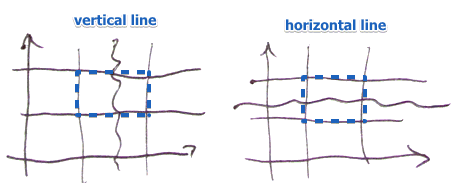# ML Wiki

## Main Idea

In each dimension, using Grid Lines, we divide our key space into stripes

•• lines divide the space into subspaces that are large enough to store one block
• if needed, overflow blocks may be used

### Representation

Each stripe points to some block on disk

•• note that we have two empty buckets there, and don't have any pointers to actual blocks
• may allow for overflow blocks

## Operations

### Lookup

• need to know values for each grid line
• so it's different from just applying a Hash Function
• we look at each component of the tuple and determine the position in the grid
• may add single-dimension index (such as B-Tree) for coordinates of each line to speed up finding the proper coordinate

### Insert

• Locate the needed bucket
• if there is room - insert
• no room - two options
• Overflow blocks
• Split by creating new grid lines (hard)

Splitting

• content of blocks is linked across dimensions
• so adding a grid line will split all the buckets along this line
•• for $n$ dimensions we may choose which dimension to split
•### Delete

• Find the corresponding bucket
• delete the record
• reorganize if needed

## Summary

Recall the typical of queries we want to answer for Multi-Dimensional Indexes

• (+) good support for
• point queries
• partial match queries (we know where to look to needed data)
• range queries
• (+) reasonable support for
• nearest neighbor queries (first look withing the bucket, then neighbor buckets and so on)
• (-) downsides
• many empty buckets when data is not uniformly distributed
• need to have a good algorithm that splits the space